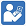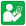Test Sheets - Multiply Decimals
Multiply Decimals - Test 1
Multiply Decimals - Test 2Practice Sheets - Multiply Decimals
Multiply Decimals - Practice 1
Multiply Decimals - Practice 2
Multiply Decimals - Practice 3